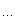# coreBugZJ

## reverse order 2

Time Limit: 1 Sec Memory Limit: 128 MB
Submissions: 157 Solved: 64

## Description

Here is a sequence a1..n, which is a disordered sequence from 1 to N. if i < j and ai > aj, <i, j> is called a pair of inversion. And b1..n-1 is defined as follows, bk is the number of the total inversion pairs in array a, when i<=k<j. Now the array a is required while the array b is known.

## Input

Several cases end with the end of the file;

And each of the cases includes two lines, a integer n(2<=n<=10^5)in the first line, and the second line followed with n-1 integer, which is in the presentation of array b;

## Output

Output the answer of each case in a line, namely the array a, and a space is required between adjacent integers.

```5
2 1 2 0```

## Sample Output

`3 1 4 2 5`
` `
`a[ 1 ] = b[ 1 ] + 1;`
`求 b[ i ] 时，a[ i ] 左边比它大的有 X 个，a[ i ] 右边比它小的有 Y 个，则比 a[ i ] 小的一共有 ( Y - X + i - 1 ) 个，所以 a[ i ] = Y - X + i，`
`即 a[ i ] = b[ i ] - b[ i - 1 ] + i。`
` `
`用 int 的 b 会错误，要 long long 的 b 。`
` `
` `
``` 1/**//* 2// int b, WA 3#include <stdio.h> 4#include <string.h> 56#define  L  100009 78int has[ L ]; 910int main() {11int n, i, a, b, bk;12while ( scanf( "%d", &n ) == 1 ) {13memset( has, 0, sizeof(has) );14bk = 0;15for ( i = 1; i < n; ++i ) {16scanf( "%d", &b );17a  = b - bk + i;18bk = b;19has[ a ] = 1;20printf( "%d ", a );21}22for ( i = 1; i <= n; ++i ) {23if( has[ i ] == 0 ) {24a = i;25}26}27printf( "%d\n", a );28}29return 0;30}31*/323334// long long b, AC35#include <stdio.h>36#include <string.h>3738#define  L  1000093940int has[ L ];4142int main(){43int n, i, a;44long long bk, b;45while ( scanf( "%d", &n ) == 1 ){46memset( has, 0, sizeof(has) );47bk = 0;48for ( i = 1; i < n; ++i ){49scanf( "%lld", &b );50a  = b - bk + i;51bk = b;52has[ a ] = 1;53printf( "%d ", a );54}55for ( i = 1; i <= n; ++i ){56if( has[ i ] == 0 ){57a = i;58}59}60printf( "%d\n", a );61}62return 0;63}64```

posted on 2011-04-10 22:57 coreBugZJ 阅读(3447) 评论(0)  编辑 收藏 引用 所属分类: ACM